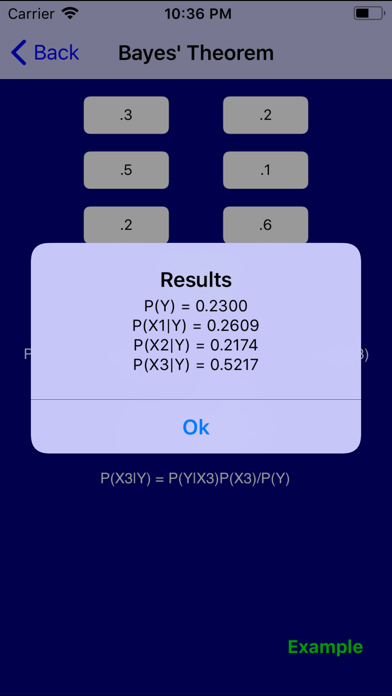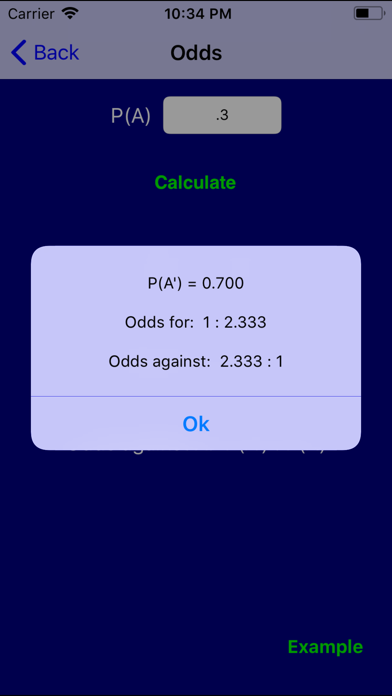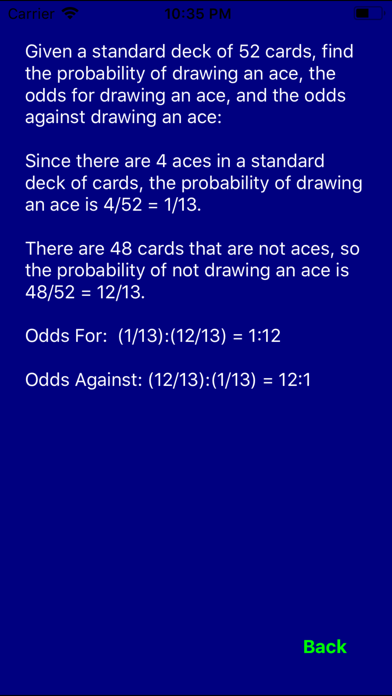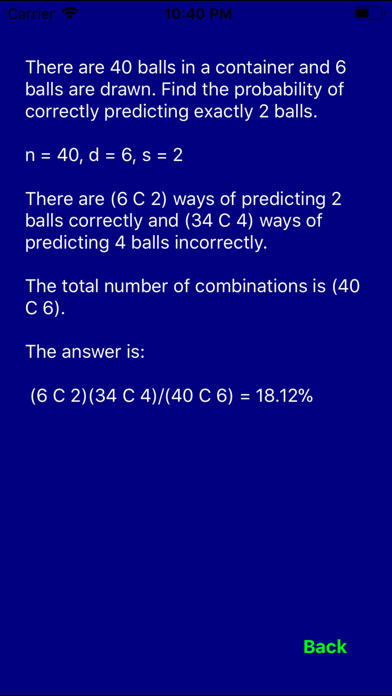# Probability Pro

## iOS UniversalEducation

\$0.99

Pop quiz: you flip a fair coin 50 times. What is the probability that it lands on heads at least 25 times?

The number of computations involved in answering that question is staggering. The formulas can cause nightmares.

"Probability Pro" will tell you that the answer is 55.61% instantly.

How about this classic? In a room of 23 people, what is the probability that at least two people share the same birthday? Believe it or not, it's 50.73%. This powerful application will explain why, and it will calculate the probability for any number of people.

How long would it take you to figure out the answers to those questions without "Probability Pro?" How much time do you want to spend checking your homework?

This application features a rich set of tutorials, examples and solvers for the following topics:

- Odds
- Survey Results
- Venn Diagrams
- Bayes' Theorem
- Permutations
- Combinations
- Taking Balls from Urns
- Replacement vs. Non-Replacement
- Independent Events
- Dice
- Roulette
- Lottery
- Poker
- General Card Problems
- Expected Value
- Birthday Problem
- Normal Distributions
- Poisson Distributions

Please note: there are multiple solvers, but there aren't solvers for every topic.

I welcome all comments, questions, and suggestions: [email protected]

Follow me on facebook for app news, tips, and free Math help:

Please check out my other applications:

ACT Math Prep
Algebra Pro
Calculus Pro
Finite Math Pro
Geometry Pro
Math Pro (six Math apps for the price of two!)
Physics Pro
PreCalculus Pro
SAT Math Prep
Statistics Pro

## What's New

- Formatting enhancements

••••••••••